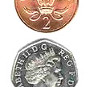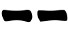# Six CoinsI learned about this method on Clarity (a well known site with lots of information and very good community forums)  which reports it to be described  in the book "I Ching Made Easy" by Rod & Amy Sorrell.

In line with the book's title, this is an easy way to cast an hexagram and proceeds as follows:

1. Take five identical coins and one coin that is notably different from the others
2. Shake the six coins and throw them on the table
3. Without looking, align them in a vertical line on the table
4. Draw a line for each coin in the order you aligned them:
• For each of the five identical coins:
• If it's head, draw;
• If it's tail, draw;
• For the different coin:
• If it's head, draw;
• If it's tail, draw;

#### Probabilites

This method always produces exactly one changing line meaning that there are only 276 possible responses (out of the 4096 that are theoretically possible).

The probabilities for each lines are:

Prob(6) = Prob(9) = 1/6 * 1/2 = 8.33%
Prob(8) = Prob(7) = 5/6 * 1/2 = 41.67%
Prob(yin) = Prob(yang) = 1/2

#### Variations

Instead of using a different coin for getting a moving line, you can throw the coins six times generating 6 hexagrams from which you can derive 6 line using the process described for the I Ching Book method.

---

I received a good suggestion from Tsukiko for another variation:
1. Take six coins (it doesn't matter if they are all equal or not)
2. Shake the six coins and throw them on the table
3. Without looking, align them in a vertical line on the table
4. Draw a line for each coin in the order you aligned them:
• If it's head, draw;
• If it's tail, draw;
5. Repeat steps 2 and 3
6. This time for each coin:
• If it's head, the corresponding line is a moving line;
• If it's tail, the corresponding line is not a moving line;
This variation gives the same probability to each line:

Prob(6) = Prob(9) = Prob(8) = Prob(7) = 1/2 * 1/2 = 1/4
Prob(yin) = Prob(yang) = 1/2

: